# Object detection for self-driving cars

In the previous blog, Introduction to Object detection, we learned the basics of object detection. We also got an overview of the YOLO (You Look Only Once algorithm). In...

In the previous blog, Introduction to Object detection, we learned the basics of object detection. We also got an overview of the YOLO (You Look Only Once algorithm). In this blog, we will extend our learning and will dive deeper into the YOLO algorithm. We will learn topics such as intersection over area metrics, non maximal suppression, multiple object detection, anchor boxes, etc. Finally, we will build an object detection detection system for a self-driving car using the YOLO algorithm. We will be using the Berkeley driving dataset to train our model.

## Data Preprocessing

Before, we get into building the various components of the object detection model, we will perform some preprocessing steps. The preprocessing steps involve resizing the images (according to the input shape accepted by the model) and converting the box coordinates into the appropriate form. Since we will be building a object detection for a self-driving car, we will be detecting and localizing eight different classes. These classes are ‘bike’, ‘bus’, ‘car’, ‘motor’, ‘person’, ‘rider’, ‘train’, and ‘truck’. Therefore, our target variable will be defined as:

where,

\begin{equation}
\hat{y} ={
\begin{bmatrix}
{p_c}& {b_x} & {b_y} & {b_h} & {b_w} & {c_1} & {c_2} & … & {c_8}
\end{bmatrix}}^T
\end{equation}

pc : Probability/confidence of an object being present in the bounding box

bx, by : coordinates of the center of the bounding box

bw : width of the bounding box w.r.t the image width

bh : height of the bounding box w.r.t the image height

ci = Probability of the ith class

But since the box coordinates provided in the dataset are in the following format: xmin, ymin, xmax, ymax (see Fig 1.), we need to convert them according to the target variable defined above. This can be implemented as follows:

W : width of the original image
H : height of the original image

\begin{equation}
b_x = \frac{(x_{min} + x_{max})}{2 * W}\ , \ b_y = \frac{(y_{min} + y_{max})}{2 * H} \\
b_w = \frac{(x_{max} – x_{min})}{2 * W}\ , \ b_y = \frac{(y_{max} + y_{min})}{2 * W}
\end{equation}

## Intersection Over Union

Intersection over Union (IoU) is an evaluation metric that is used to measure the accuracy of an object detection algorithm. Generally, IoU is a measure of the overlap between two bounding boxes. To calculate this metric, we need:

• The ground truth bounding boxes (i.e. the hand labeled bounding boxes)
• The predicted bounding boxes from the model

Intersection over Union is the ratio of the area of intersection over the union area occupied by the ground truth bounding box and the predicted bounding box. Fig. 9 shows the IoU calculation for different bounding box scenarios.

Intersection over Union is the ratio of the area of intersection over the union area occupied by the ground truth bounding box and the predicted bounding box. Fig. 2 shows the IoU calculation for different bounding box scenarios.

Now, that we have a better understanding of the metric, let’s code it.

## Defining the Model

Instead of building the model from scratch, we will be using a pre-trained network and applying transfer learning to create our final model. You only look once (YOLO) is a state-of-the-art, real-time object detection system, which has a mAP on VOC 2007 of 78.6% and a mAP of 48.1% on the COCO test-dev. YOLO applies a single neural network to the full image. This network divides the image into regions and predicts the bounding boxes and probabilities for each region. These bounding boxes are weighted by the predicted probabilities.

One of the advantages of YOLO is that it looks at the whole image during the test time, so its predictions are informed by global context in the image. Unlike R-CNN, which requires thousands of networks for a single image, YOLO makes predictions with a single network. This makes this algorithm extremely fast, over 1000x faster than R-CNN and 100x faster than Fast R-CNN.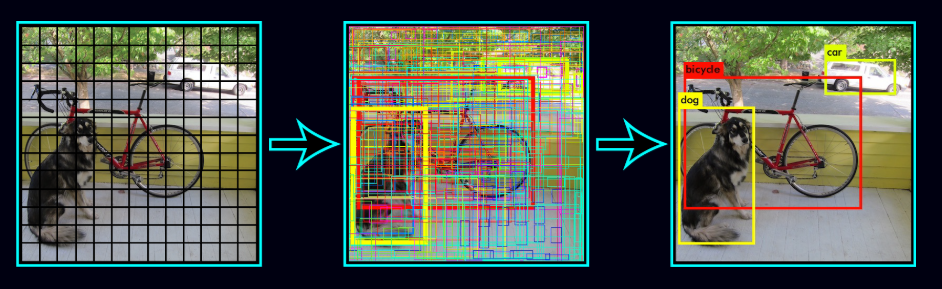### Loss Function

If the target variable $# y$#  is defined as

\begin{equation}
y ={
\begin{bmatrix}
{p_c}& {b_x} & {b_y} & {b_h} & {b_w} & {c_1} & {c_2} & {…} & {c_8}
\end{bmatrix}}^T \\
\begin{matrix}
& {y_1}& {y_2} & {y_3} & {y_4} & {y_5} & {y_6} & {y_7} & {…} & {y_{13}}
\end{matrix}
\end{equation}

the loss function for object localization is defined as

\begin{equation}
\mathcal{L(\hat{y}, y)} =
\begin{cases}
(\hat{y_1} – y_1)^2 + (\hat{y_2} – y_2)^2 + … + (\hat{y_{13}} – y_{13})^2 &&, y_1=1 \\
(\hat{y_1} – y_1)^2 &&, y_1=0
\end{cases}
\end{equation}

The loss function in case of the YOLO algorithm is calculated using the following steps:

• Find the bounding boxes with the highest IoU with the true bounding boxes
• Calculate the confidence loss (the probability of object being present inside the bounding box)
• Calculate the classification loss (the probability of class present inside the bounding box)
• Calculate the coordinate loss for the matching detected boxes.
• Total loss is the sum of the confidence loss, classification loss, and coordinate loss.

Using the steps defined above, let’s calculate the loss function for the YOLO algorithm.

In general, the target variable is defined as

\begin{equation}
y ={
\begin{bmatrix}
{p_i(c)}& {x_i} & {y_i} & {h_i} & {w_i} & {C_i}
\end{bmatrix}}^T
\end{equation}

where,

pi(c) : Probability/confidence of an object being present in the bounding box.
xi, yi : coordinates of the center of the bounding box.
wi : width of the bounding box w.r.t the image width.
hi : height of the bounding box w.r.t the image height.
Ci = Probability of the ith class.

then the corresponding loss function is calculated as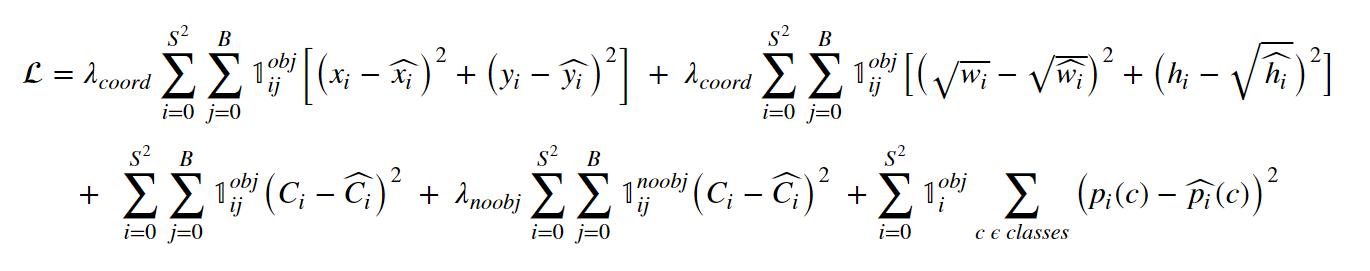where,The above equation represents the yolo loss function. The equation may seem daunting at first, but on having a closer look we can see it is the sum of the coordinate loss, the classification loss, and the confidence loss in that order. We use sum of squared errors because it is easy to optimize. However, it weights the localization error equally with classification error which may not be ideal. To remedy this, we increase the loss from bounding box coordinate predictions and decrease the loss from confidence predictions for boxes that don’t contain objects. We use two parameters, λcoord and λnoobj to accomplish this.

Note that the loss function only penalizes classification error if an object is present in that grid cell. It also penalizes the bounding box coordinate error if that predictor is responsible for the ground truth box (i.e which has the highest IOU of any predictor in that grid cell).

### Model Architecture

The YOLO model has the following architecture (see Fig 3). The network has 24 convolutional layers followed by two fully connected layers. Alternating 1 × 1 convolutional layers reduce the features space from preceding layers. The convolutional layers are pretrained on the ImageNet classification task at half the resolution (224 × 224 input image) and then double the resolution for detection.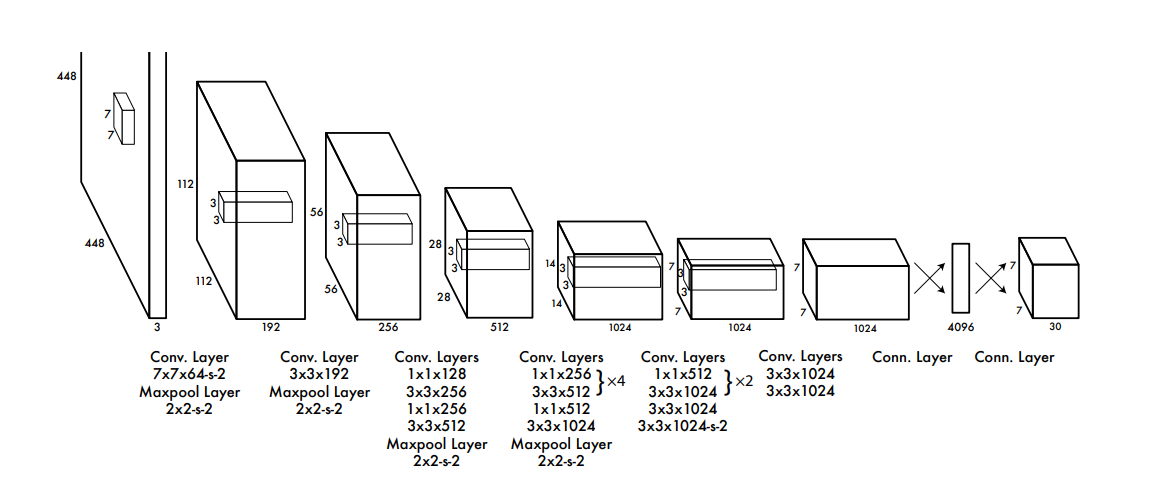Fig 3. The YOLO Archtecture (Image taken from the official YOLO paper)

We will be using pre trained YOLOv2 model, which has been trained on the COCO image dataset with classes similar to the Berkeley Driving Dataset. So, we will use the YOLOv2 pretrained network as a feature extractor. We will load the pretrained weights of the YOLOv2 model and will freeze all the weights except for the last layer during training of the model. We will remove the last convolutional layer of the YOLOv2 model and replace it with a new convolutional layer indicating the number of classes (8 classes as defined earlier) to be predicted. This is implemented in the following code.

Due to limited computational power, we used only the first 1000 images present in the training dataset to train the model. Finally, we trained the model for 20 epochs and saved the model weights with the lowest loss.

## Tackling Multiple Detection

### Threshold Filtering

The YOLO object detection algorithm will predict multiple overlapping bounding boxes for a given image. As not all bounding boxes contain the object to be classified (e.g. pedestrian, bike, car or truck) or detected, we need to filter out those bounding boxes that don’t contain the target object. To implement this, we monitor the value of pc, i.e., the probability or confidence of an object (i.e. the four classes) being present in the bounding box. If the value of pc is less than the threshold value, then we filter out that bounding box from the predicted bounding boxes. This threshold may vary from model to model and serve as a hyper-parameter for the model.

If predicted target variable is defined as:

\begin{equation}
\hat{y} ={
\begin{bmatrix}
{p_c}& {b_x} & {b_y} & {b_h} & {b_w} & {c_1} & {c_2} & … & {c_8}
\end{bmatrix}}^T
\end{equation}

then discard all bounding boxes where the value of pc < threshold value. The following code implements this approach.

### Non-max Suppression

Even after filtering by thresholding over the classes score, we may still end up with a lot of overlapping bounding boxes. This is because the YOLO algorithm may detect an object multiple times, which is one of its drawbacks. A second filter called non-maximal suppression (NMS) is used to remove duplicate detections of an object. Non-max suppression uses ‘Intersection over Union’ (IoU) to fix multiple detections.

Non-maximal suppression is implemented as follows:

• Find the box confidence (pc) (Probability of the box containing the object) for each detection.
• Pick the bounding box with the maximum box confidence. Output this box as prediction.
• Discard any remaining bounding boxes which have an IoU greater than 0.5 with the bounding box selected as output in the previous step i.e. any bounding box with high overlap is discarded.

In case there are multiple classes/ objects, i.e., if there are four objects/classes, then non-max suppression will run four times, once for every output class.

### Anchor boxes

One of the drawbacks of YOLO algorithm is that each grid can only detect one object. What if we want to detect multiple distinct objects in each grid. For example, if two objects or classes are overlapping and share the same grid as shown in the image (see Fig 4.),

We make use of anchor boxes to tackle the issue. Let’s assume the predicted variable is defined as

\begin{equation}
\hat{y} ={
\begin{bmatrix}
{p_c}& {b_x} & {b_y} & {b_h} & {b_w} & {c_1} & {c_2} & {…} & {c_8}
\end{bmatrix}}^T
\end{equation}

then, we can use two anchor boxes in the following manner to detect two objects in the image simultaneously.

Earlier, the target variable was defined such that each object in the training image is assigned to grid cell that contains that object’s midpoint. Now, with two anchor boxes, each object in the training images is assigned to a grid cell that contains the object’s midpoint and anchor box for the grid cell with the highest IOU. So, with the help of two anchor boxes, we can detect at most two objects simultaneously in an image. Fig 6. shows the shape of the final output layer with and without the use of anchor boxes.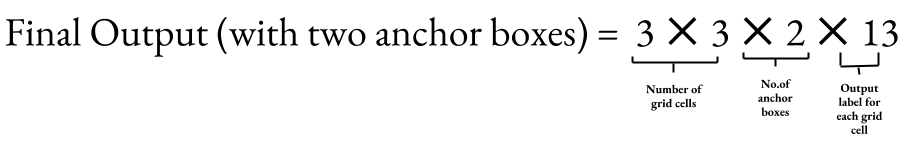Fig 6. Shape of the output layer with two anchor boxes

Although, we can detect multiple images using Anchor boxes, but they still have limitations. For example, if there are two anchor boxes defined in the target variable and the image has three overlapping objects, then the algorithm fails to detect all three objects. Secondly, if two anchor boxes are associated with two objects but have the same midpoint in the box coordinates, then the algorithm fails to differentiate between the objects. Now, that we know the basics of anchor boxes, let’s code it.

In the following code we will use 10 anchor boxes. As a result, the algorithm can detect at maximum of 10 objects in a given image.

We can combine both the concepts threshold filtering and non-maximal suppression and apply it on the output predicted by the YOLO model. This is implemented in the code below.

## Object Detection on Sample Test Image

We will use the trained model to predict the respective classes and the corresponding bounding boxes on a sample of images. The function ‘draw’ runs a tensorflow session and calculates the confidence scores, bounding box coordinates and the output class probabilities for the given sample image. Finally, it computes the xmin, xmax, ymin, ymax from bx,by,bw,bh, scales the bounding boxes according to the input sample image and draws the bounding boxes and class probability for the objects in the input sample image.

#### Fig. 7, shows the class probabilities and bounding boxes on the test images.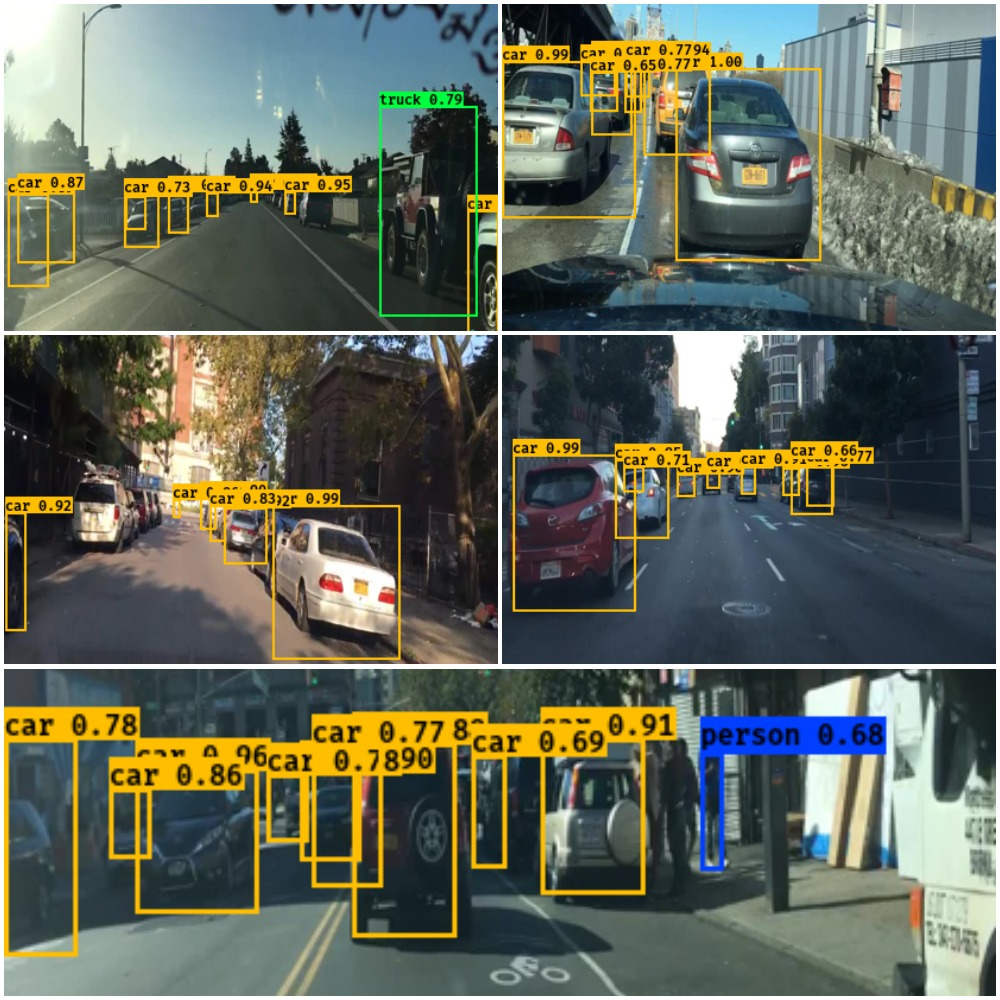Fig 7. Sample images with the predicted classes and bounding boxes

## Implementing the Model on Real Time Video

Next, we will implement the model on a real time video. Since, video is a sequence of images at different time frames, so we will predict the class probabilities and bounding boxes for the image captured at each time frame. We will use OpenCV video capture function to read the video and convert it into image/ frames at different time steps. The video below demonstrates the implementation of the algorithm on a real time video.

[Source Code]

## Conclusion

This brings us to the end of this article. Congratulate yourself on reaching to the end of this blog. As a reward you now have a better understanding of how object detection works (using the YOLO algorithm) and how self driving cars implement this technique to differentiate between cars, trucks, pedestrians, etc. to make better decisions. Finally, I encourage you to implement and play with the code yourself. You can find the full source code related to this article here.

Have anything to say? Feel free to comment below for any questions, suggestions, and discussions related to this article. Till then, keep hacking with HackerEarth.

Struggling to compose your own music, check out this blog on how to Compose Jazz Music with Deep Learning.

### References0 Followers

Most reacted commentRecent comment authors